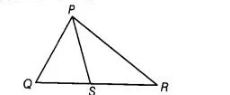# S is any point on side QR of a ΔPQR. Show that PQ + QR + RP > 2 PS.`
Question:

S is any point on side QR of a ΔPQR. Show that PQ + QR + RP > 2 PS.

Thinking Process

Use the inequality of a triangle i.e., sum of two sides of a triangle is greater than the third side. Further, show the required result.

Solution:

Given In $\triangle P Q R, S$ is any point on side $Q R$.To show $P Q+Q R+R P>2 P S$

Proof $\ln \triangle P Q S$, ... (i)

[sum of two sides of a triangle is greater than the third side]

Similarly, in $\triangle P R S$, $S R+R P>P S$.....(ii)

[sum of two sides of a triangle is greater than the third side]

On adding Eqs. (1) and (ii), we get

$P Q+Q S+S R+R P>2 P S$

$\Rightarrow \quad P Q+(Q S+S R)+R P>2 P S$

$\Rightarrow \quad P Q+Q R+R P>2 P S \quad[\because Q R=Q S+S R]$